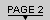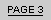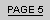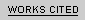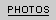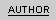M O D E R N I T Y   A N D   T H E   M A N I A CP a g e   T w o :   J a n e t   L e i g h   i n   T o u c h   o f   E v i l " I   w a s   j u s t   l i s t e n i n g   t o   y o u   b r e a t h e , "   s a y s   S u s i e   t o   h e r   h u s b a n d .A R T I C L E   B Y   R I C H A R D   A R M S T R O N G